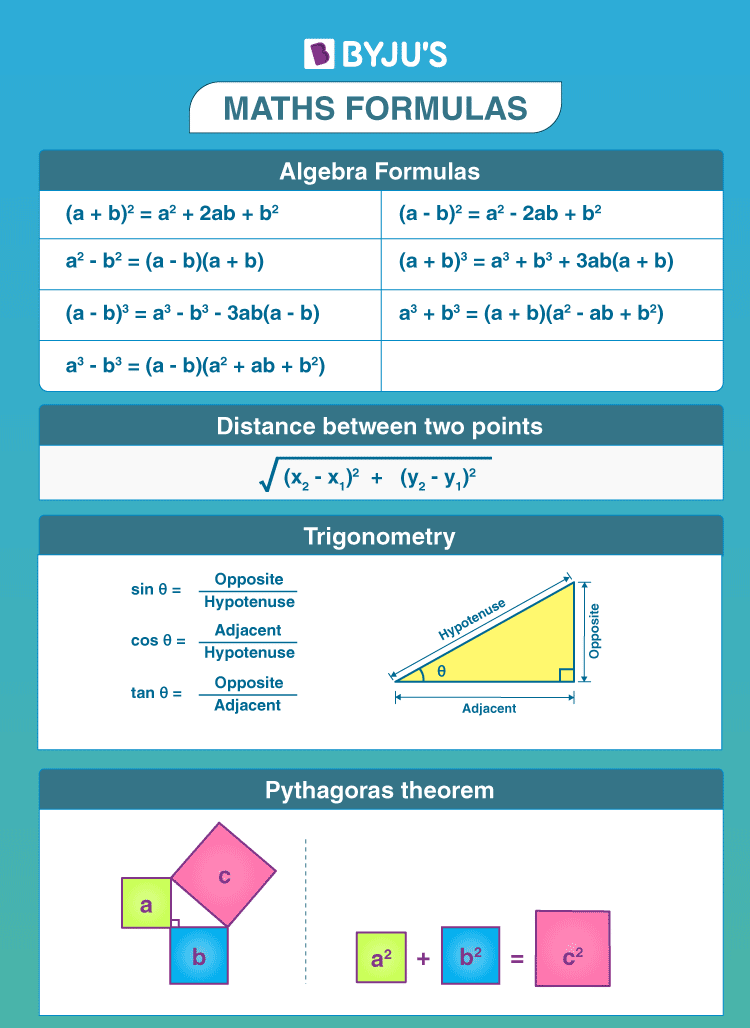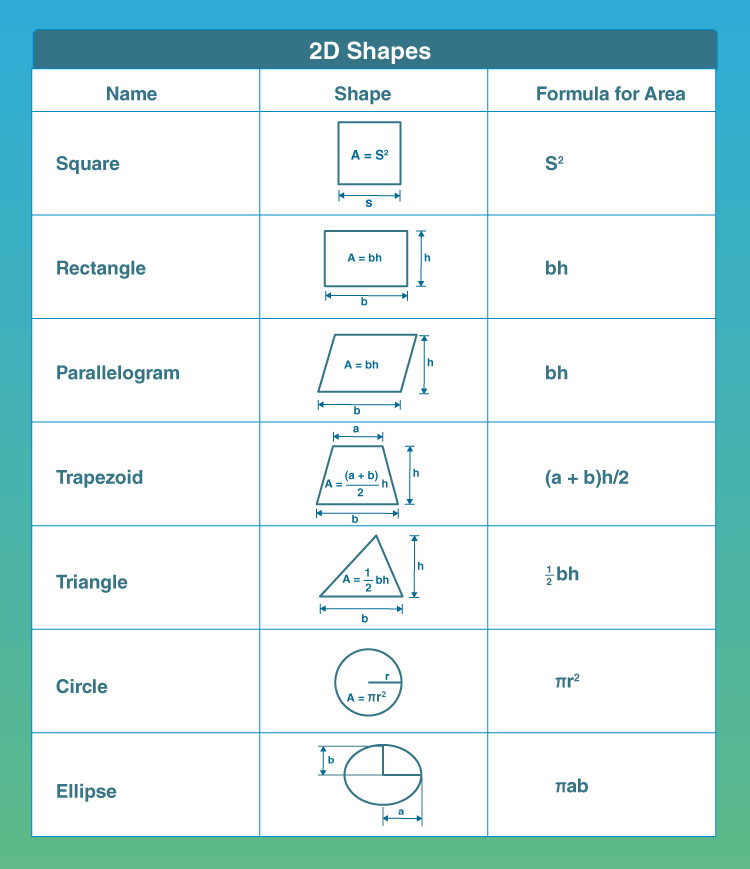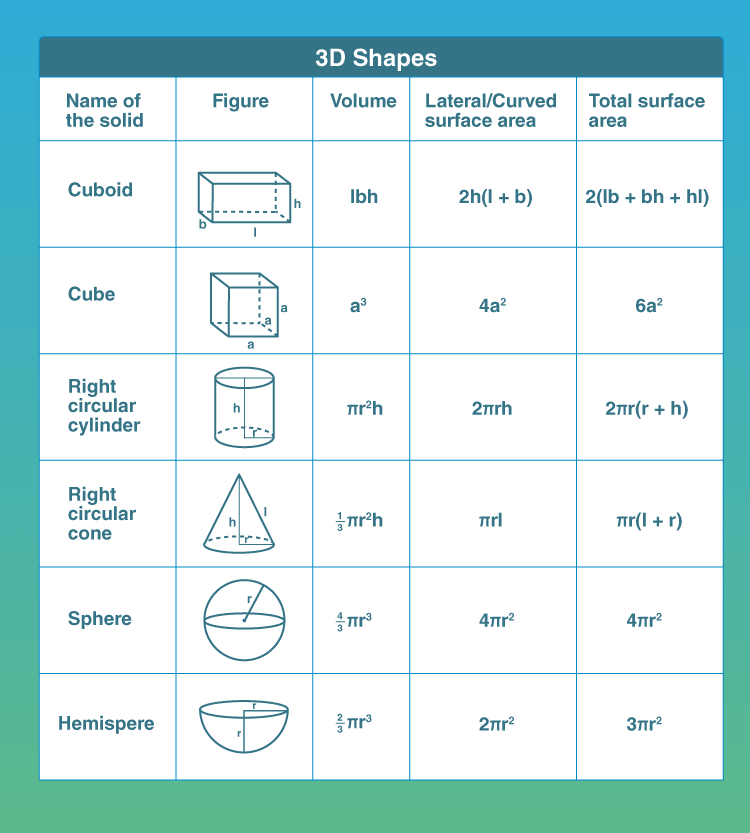# Math Formulas

Maths formulas, for all the concepts covered under different classes (6, 7, 8, 9, 10, 11, and 12), as per the CBSE syllabus are provided here by our expert teachers. To solve the mathematical problems easily, students should learn and remember the basic formulas based on certain fundamentals such as algebra, arithmetic, and geometry. Also, check with Maths Syllabus here for all classes.

Addition, subtraction, multiplication and division are easy; what if you come across derivation, calculus and geometry? You would need formulas to solve them. Here, at BYJU’S, you get a unique way of solving the maths problem, which will make you learn how the equation came into existence. This is the better way of memorizing and applying the formulas.

We present you with a host of formulas (more than 400) for your reference to solve all important mathematical operations and questions. Also, each formula here is given with solved examples.

## List of Maths Formulas (for All Concepts)

The above given formulas are very helpful for students to solve problems based on them. All the formulas are also provided here, along with solved examples to help you understand the application of formulas.

See the Maths videos here for a more comprehensive approach to solve maths problems using formulas.

Feel free to use our directory of formulas for your homework.

## Basic Maths Formulas

The Math Formula Chart has all the basic math formulas, below:### Formulas table

Go through the table given below to learn all the basic maths formulas.

 Perimeter Square Rectangle P = 4a P = 2(l+b) Circumference Circle C = 2 (pi) r Area Square Rectangle Triangle Trapezoid Circle A = a2 A = l x b A = ½(b x h) A = ((b1 +b2 ) x h) / 2 A = π x r 2 Surface Area Cube Cylinder Cone Sphere S = 6l2 CSA = 2 x π x r x h CSA = π x r x l S = 4 x π x r 2 Volume Cylinder Cone Sphere V = πr 2h V =1/3 πr 2h V = 4/3 x π x r3 Pythagoras Theorem a2 + b2 = c2 Distance Formula d = √[(x2 – x1)2 +(y2 – y1)2] Slope of a line m = y2 – y1 / x2 – x1 Mid- Point Formula M = [(x1 + x2 )/ 2 , (y1 + y2 )/ 2] Algebraic Formula Pythagorean theorem Slope-intercept form of the equation of a line Distance formula Total cost Quadratic formula Laws of Exponents Fractional Exponents a2 + b2 = c2 y = mx + c d = rt total cost = (number of units) × (price per unit) X = [-b ± √(b2 – 4ac)] /2a am x b m = (a x b)m; am x a n = (a)m+n a1/2 = √a Trigonometric Formulas Sine Function Cosine Function Tangent Function Sin x = Opposite Side/ Hypotenuse Cos X = Adjacent Side/ Hypotenuse Tan x = Opposite Side/ Adjacent Side

Additional guidelines based on all Math formulas are given on our site. Students can also get help from our tutors who are available 24/7.

## Frequently Asked Questions – FAQs

Q1

### What are the basic Maths formulas?

The basic Maths formulas include arithmetic operations, where we learn to add, subtract, multiply and divide. Also, algebraic identities help to solve equations. Some of the formulas are:
(a + b)2 = a+ b+ 2ab
(a – b)2 = a+ b– 2ab
a– b2 = (a + b) (a – b)
Q2

### What are the fundamental topics for formulas in Maths?

The fundamental concepts we learn for maths formulas are:
Area and perimeter of shapes
Surface areas and Volumes
Algebra
Powers and exponents
Fractions
Percentage, etc.
Q3

### What are the important Maths formulas?

The important formulas are related to algebra, Pythagoras theorem, series and sequence, mensuration, calculus, probability and statistics, trigonometry, matrices, etc.
Q4

### What are the area and perimeter formulas?

The area of a shape defines the region covered by it whereas the perimeter gives the total length of the outer boundary of the shape. The area is measured in square units but the perimeter is denoted by the unit of the dimension. Suppose, the formula for the area of a square is side2 sq. units. and perimeter of a square is 4(side) units.
Q5

### What is a3 – b3 formula?

The formula for a– b3 is given by:
a– b3 = (a – b) (a+ ab + b2 )

1. Very helful

2. i am very proud to use this website

3. Really it is a very useful app for everyone .

4. I am very proud

5. Love this website!

6. this website is extremely Helpful

7. It help me to Solve complex Mathematics question

9. love it

10. This is the best website in the world.

11. It help me to solve complex physics question. very helpful website

12. this website is very nice